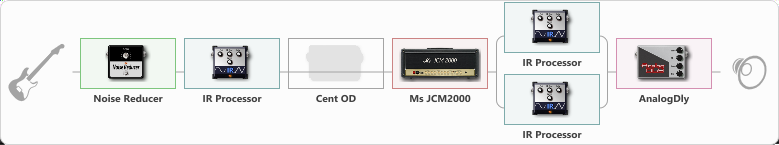# crunch

Discussion in 'ToneLib-GFX presets' started by carlinos3, Oct 14, 2021.

1. crunch

Preset name: carlos--3

Effects chain:Effect: "Noise Reducer" (Dinámica / Filtro), active - "yes"
{
"Sens" = 72
"Mode" = Hard
}

Effect: "IR Processor" (Gabinetes), active - "yes"
{
"IR" = PRE-1_dc
"Low Cut (Hz)" = 0
"Hi Cut (kHz)" = 20.0
"Mix" = 77
"Level (dB)" = 1
}

Effect: "Cent OD" (Overdrive / Distorsión), active - "no"
{
"Drive" = 38
"Tone" = 76
"Level" = 38
}

{
"Gain" = 42
"Bass" = 50
"Middle" = 50
"Treble" = 50
"Presence" = 50
"Master" = 69
"Level (dB)" = 0
}

Effect: "Splitter" (Dinámica / Filtro)
{
"A-Bypass" = Off
"A-Pan" = 62
"A-Level" = 55
"B-Bypass" = Off
"B-Pan" = -76
"B-Level" = 55

'A' branch:
{

Effect: "IR Processor" (Gabinetes), active - "yes"
{
"IR" = charv.1_dc
"Low Cut (Hz)" = 0
"Hi Cut (kHz)" = 20.0
"Mix" = 100
"Level (dB)" = 0
}
}
'B' branch:
{

Effect: "IR Processor" (Gabinetes), active - "yes"
{
"IR" = GreenDayDookie
"Low Cut (Hz)" = 0
"Hi Cut (kHz)" = 20.0
"Mix" = 100
"Level (dB)" = 0
}
}
}

Effect: "AnalogDly" (Retrasar), active - "yes"
{
"Time" = 532
"Feedback" = 0
"Tone" = 65
"Mix" = 35
}

Note: You will need to download and install the ToneLib-GFX software to use the preset.

#### Attached Files:

• ###### crunch.tlgfx
File size:
70.3 KB
Views:
1,942
alwxrz and Ass like this.